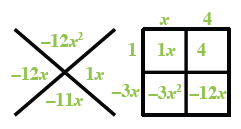### Home > AC > Chapter 13 > Lesson 13.RE2-S > Problem2-140

2-140.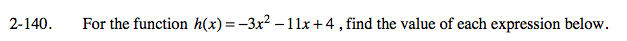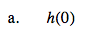Substitute 0 for x and simplify.

h(0) = −3(0)2 − 11(0) + 4

h(0) = 4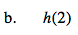See part (a).

h(2) = −30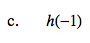Be careful of the signs when multiplying negative numbers.

h(−1) = 12Remember how to multiply fractions.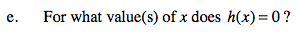0 = −3x2 − 11x + 4